# Chiang Wainwright Mathematical Economics Chapter 4 Linear Models

• Slides: 34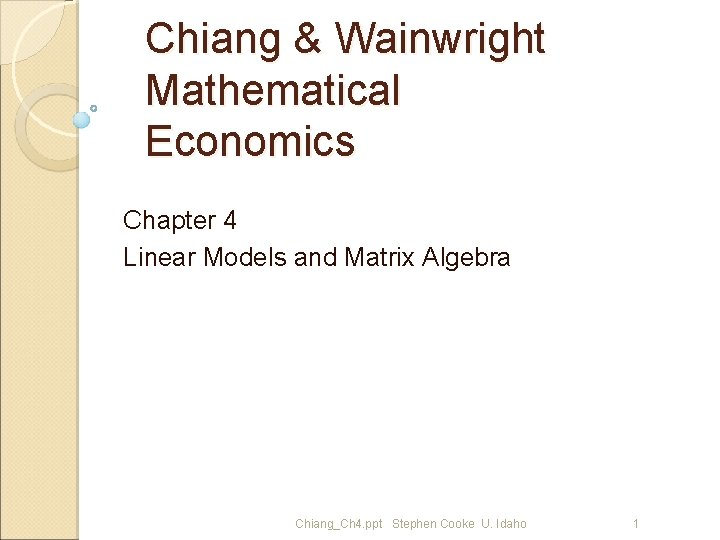Chiang & Wainwright Mathematical Economics Chapter 4 Linear Models and Matrix Algebra Chiang_Ch 4. ppt Stephen Cooke U. Idaho 1Ch 4 Linear Models and Matrix Algebra 4. 1 Matrices and Vectors 4. 2 Matrix Operations 4. 3 Notes on Vector Operations 4. 4 Commutative, Associative, and Distributive Laws 4. 5 Identity Matrices and Null Matrices 4. 6 Transposes and Inverses 4. 7 Finite Markov Chains Chiang_Ch 4. ppt Stephen Cooke U. Idaho 2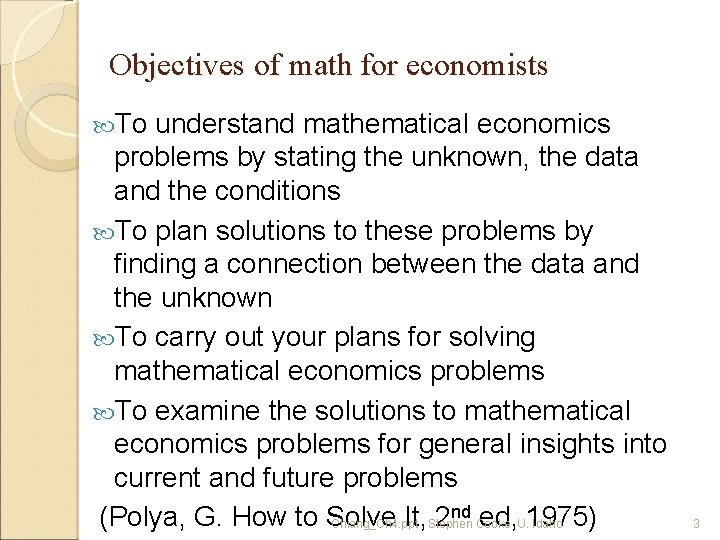Objectives of math for economists To understand mathematical economics problems by stating the unknown, the data and the conditions To plan solutions to these problems by finding a connection between the data and the unknown To carry out your plans for solving mathematical economics problems To examine the solutions to mathematical economics problems for general insights into current and future problems nd ed, 1975) (Polya, G. How to Solve It, 2 Chiang_Ch 4. ppt Stephen Cooke U. Idaho 3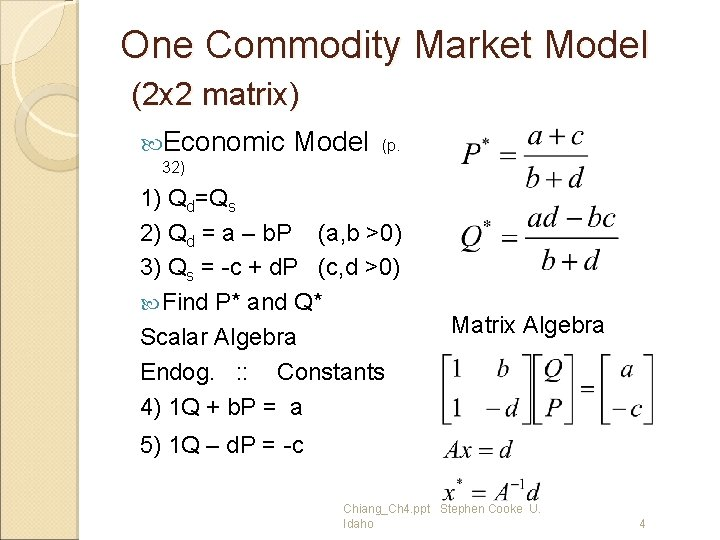One Commodity Market Model (2 x 2 matrix) Economic Model (p. 32) 1) Qd=Qs 2) Qd = a – b. P (a, b >0) 3) Qs = -c + d. P (c, d >0) Find P* and Q* Scalar Algebra Endog. : : Constants 4) 1 Q + b. P = a Matrix Algebra 5) 1 Q – d. P = -c Chiang_Ch 4. ppt Stephen Cooke U. Idaho 4One Commodity Market Model (2 x 2 matrix) Matrix algebra Chiang_Ch 4. ppt Stephen Cooke U. Idaho 5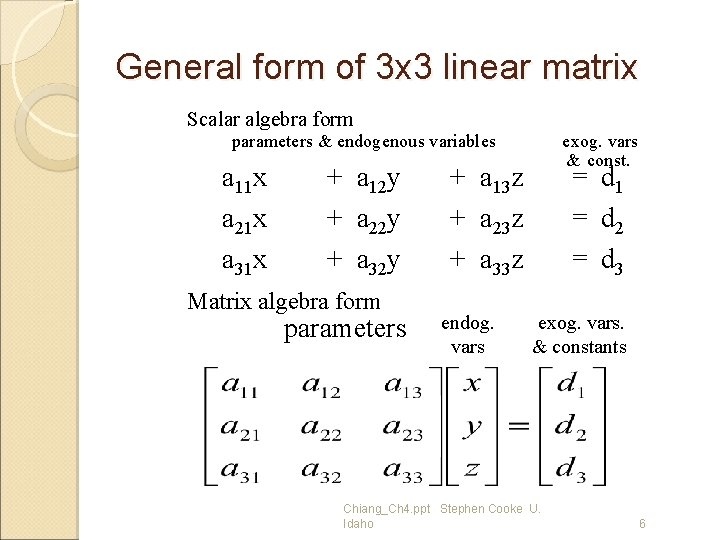General form of 3 x 3 linear matrix Scalar algebra form parameters & endogenous variables a 11 x a 21 x a 31 x + a 12 y + a 22 y + a 32 y Matrix algebra form parameters exog. vars & const. = d 1 + a 13 z + a 23 z + a 33 z endog. vars = d 2 = d 3 exog. vars. & constants Chiang_Ch 4. ppt Stephen Cooke U. Idaho 61. Three Equation National Income Model (3 x 3 matrix) Let (Exercise 3. 5 -1, p. 47) Y = C + I 0 + G 0 C = a + b(Y-T) T = d + t. Y (a > 0, 0<b<1) (d > 0, 0<t<1) Endogenous variables? Exogenous variables? Constants? Parameters? Why restrictions on the parameters? Chiang_Ch 4. ppt Stephen Cooke U. Idaho 7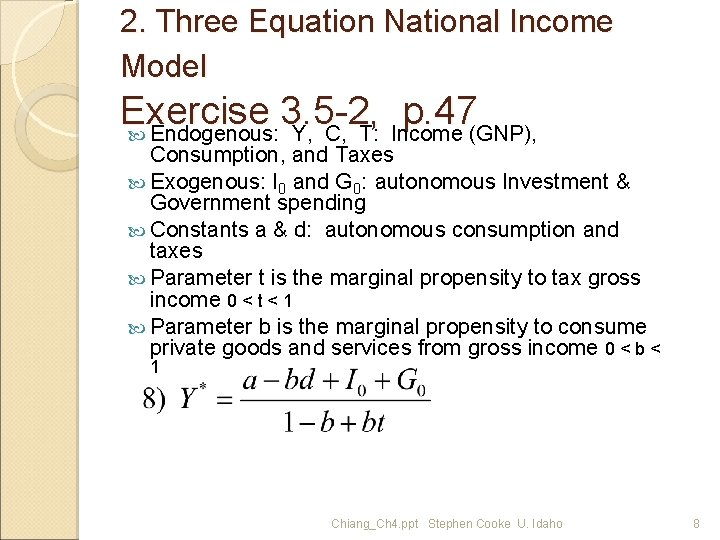2. Three Equation National Income Model Exercise 3. 5 -2, p. 47 Endogenous: Y, C, T: Income (GNP), Consumption, and Taxes Exogenous: I 0 and G 0: autonomous Investment & Government spending Constants a & d: autonomous consumption and taxes Parameter t is the marginal propensity to tax gross income 0 < t < 1 Parameter b is the marginal propensity to consume private goods and services from gross income 0 < b < 1 Chiang_Ch 4. ppt Stephen Cooke U. Idaho 86. Three Equation National Income Model Exercise 3. 5 -1 p. 47 Parameters & Given Endogenous vars. Exog. vars. Y &cons. C T Y = C + I 0 + G 0 1 Y -1 C +0 T = I 0+G 0 C = a + b(Y-T) -b. Y +1 C +b. T = a T = d + t. Y -t. Y +0 C +1 T = d Find Y*, C*, T* Chiang_Ch 4. ppt Stephen Cooke U. Idaho 10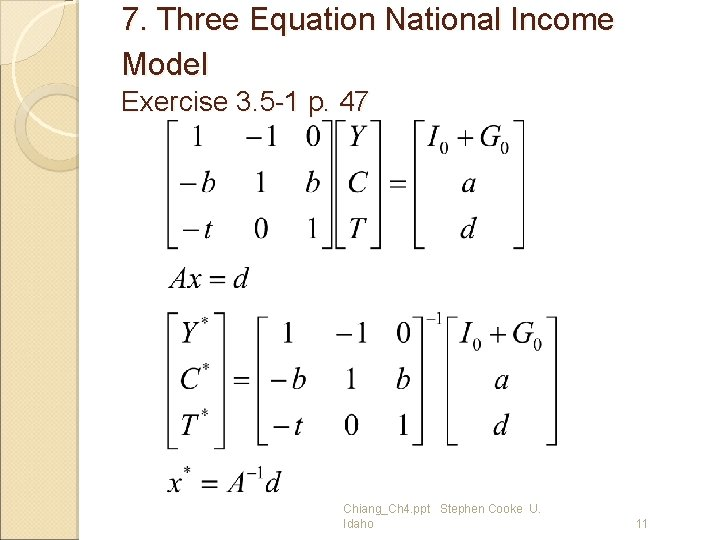7. Three Equation National Income Model Exercise 3. 5 -1 p. 47 Chiang_Ch 4. ppt Stephen Cooke U. Idaho 113. Two Commodity Market Equilibrium Section 3. 4, p. 42 Given Qdi = Qsi, i=1, 2 Qd 1 = 10 - 2 P 1 + P 2 Qs 1 = -2 + 3 P 1 Qd 2 = 15 + P 1 - P 2 Qs 2 = -1 + 2 P 2 Find Q 1*, Q 2*, P 1*, P 2* Scalar algebra 1 Q 1 +0 Q 2 +2 P 1 - 1 P 2 = 10 1 Q 1 +0 Q 2 - 3 P 1 +0 P 2= -2 0 Q 1+ 1 Q 2 - 1 P 1 + 1 P 2= 15 0 Q 1+ 1 Q 2 +0 P 1 - 2 P 2= -1 Chiang_Ch 4. ppt Stephen Cooke U. Idaho 124. Two Commodity Market Equilibrium Section 3. 4, p. 42 (4 x 4 matrix) Chiang_Ch 4. ppt Stephen Cooke U. Idaho 134. 1 Matrices and Vectors Matrices as Arrays Vectors as Special Matrices Assume an economic model as system of linear equations in which aij parameters, where i = 1. . n rows, j = 1. . m columns, and n=m xi endogenous variables, di exogenous variables and constants Chiang_Ch 4. ppt Stephen Cooke U. Idaho 14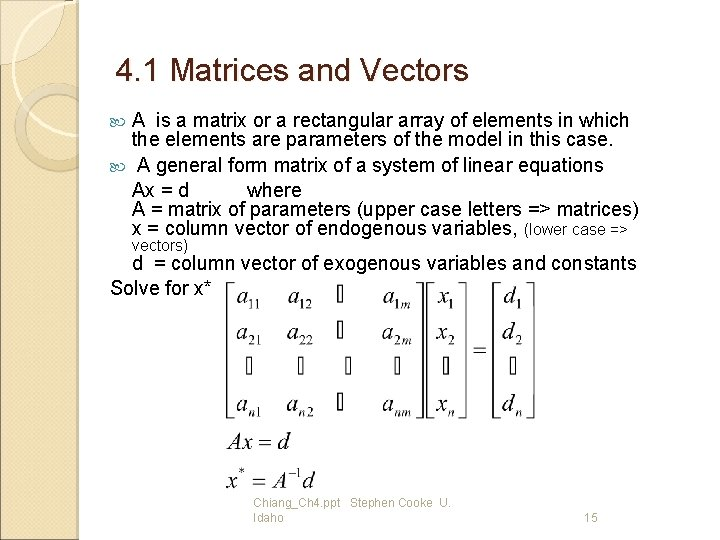4. 1 Matrices and Vectors A is a matrix or a rectangular array of elements in which the elements are parameters of the model in this case. A general form matrix of a system of linear equations Ax = d where A = matrix of parameters (upper case letters => matrices) x = column vector of endogenous variables, (lower case => vectors) d = column vector of exogenous variables and constants Solve for x* Chiang_Ch 4. ppt Stephen Cooke U. Idaho 15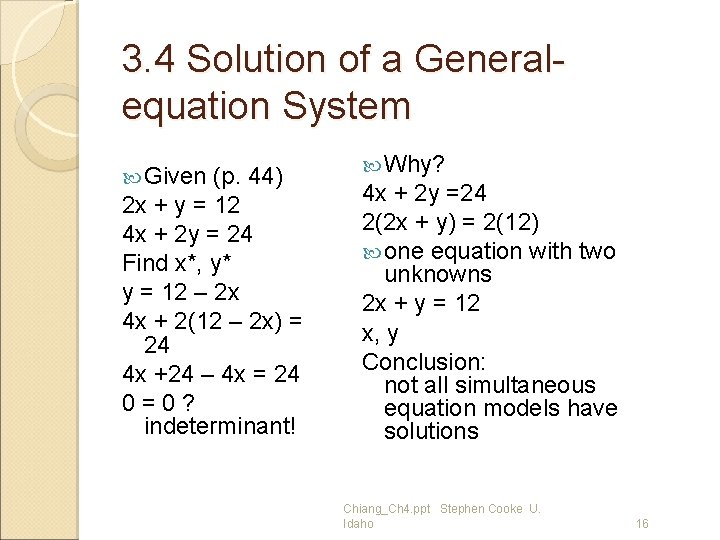3. 4 Solution of a Generalequation System Given (p. 44) 2 x + y = 12 4 x + 2 y = 24 Find x*, y* y = 12 – 2 x 4 x + 2(12 – 2 x) = 24 4 x +24 – 4 x = 24 0 = 0 ? indeterminant! Why? 4 x + 2 y =24 2(2 x + y) = 2(12) one equation with two unknowns 2 x + y = 12 x, y Conclusion: not all simultaneous equation models have solutions Chiang_Ch 4. ppt Stephen Cooke U. Idaho 16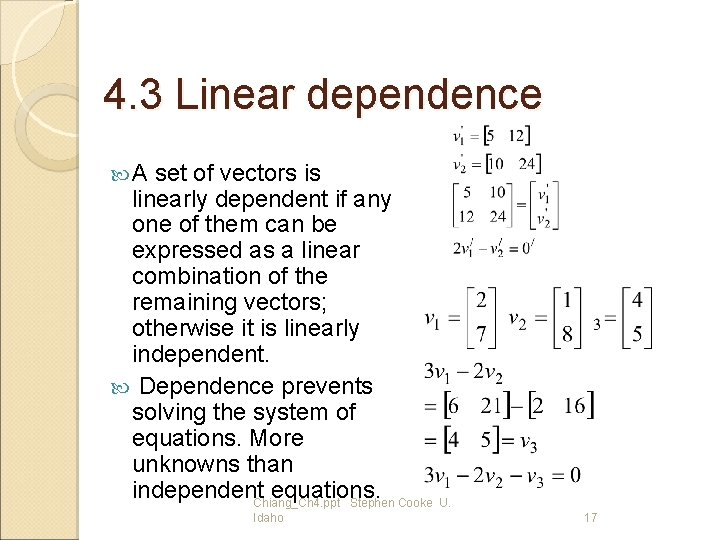4. 3 Linear dependence A set of vectors is linearly dependent if any one of them can be expressed as a linear combination of the remaining vectors; otherwise it is linearly independent. Dependence prevents solving the system of equations. More unknowns than independent equations. Chiang_Ch 4. ppt Stephen Cooke U. Idaho 17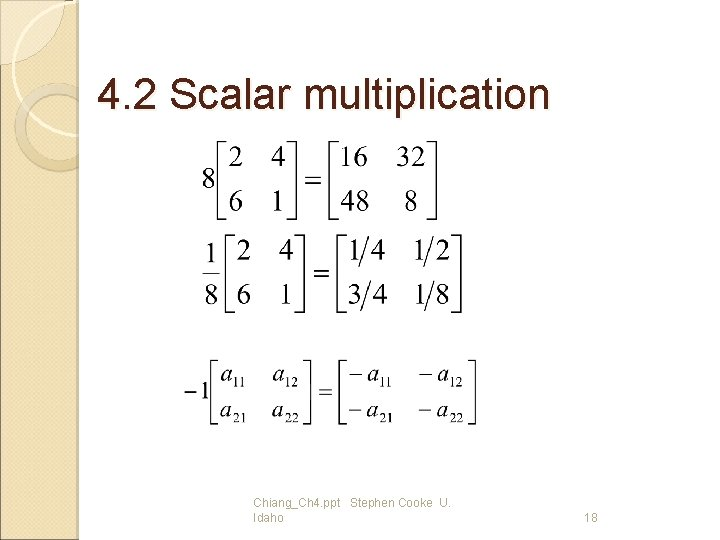4. 2 Scalar multiplication Chiang_Ch 4. ppt Stephen Cooke U. Idaho 18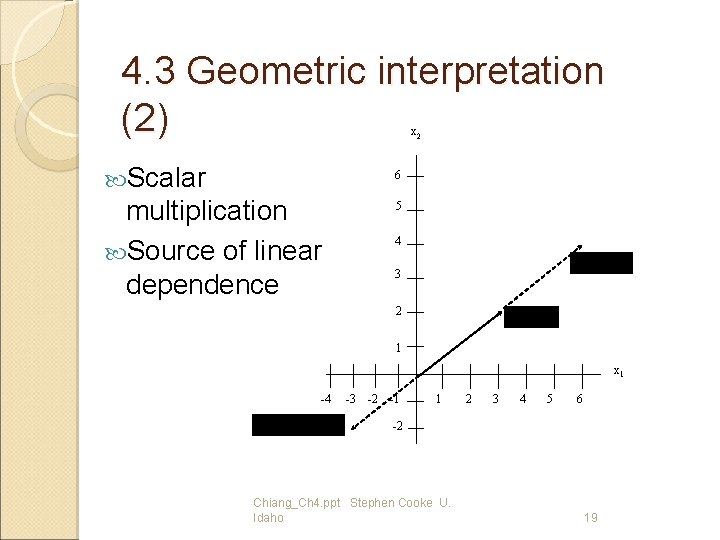4. 3 Geometric interpretation (2) x 2 Scalar 6 multiplication Source of linear dependence 5 4 3 2 1 x 1 -4 -3 -2 -1 1 2 3 4 5 6 -2 Chiang_Ch 4. ppt Stephen Cooke U. Idaho 19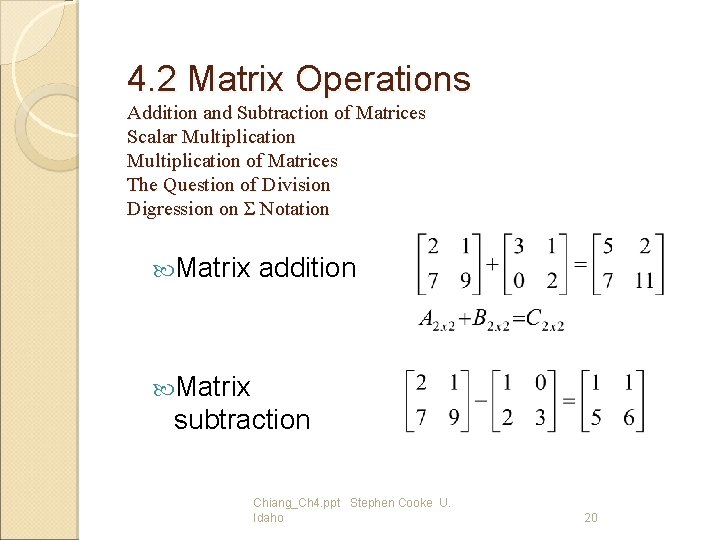4. 2 Matrix Operations Addition and Subtraction of Matrices Scalar Multiplication of Matrices The Question of Division Digression on Σ Notation Matrix addition Matrix subtraction Chiang_Ch 4. ppt Stephen Cooke U. Idaho 20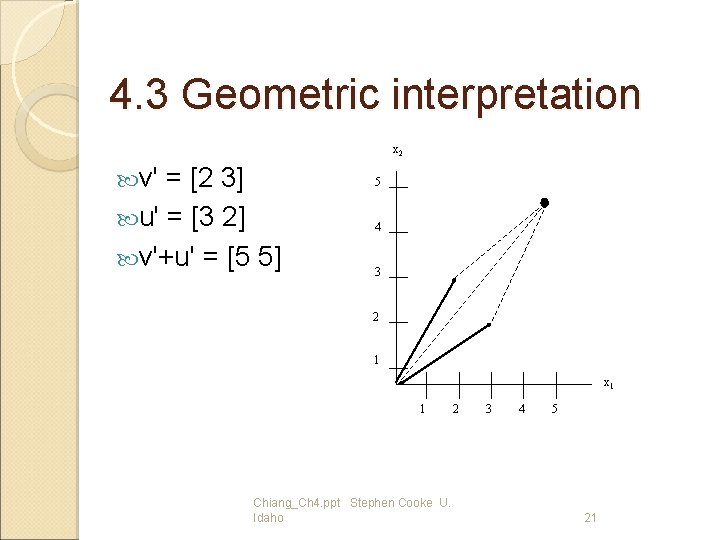4. 3 Geometric interpretation x 2 v' = [2 3] 5 u' = [3 2] 4 v'+u' = [5 5] 3 2 1 x 1 1 2 Chiang_Ch 4. ppt Stephen Cooke U. Idaho 3 4 5 214. 4 Matrix multiplication Exceptions AB=BA iff B = a scalar, B = identity matrix I, or B = the inverse of A, i. e. , A-1 Chiang_Ch 4. ppt Stephen Cooke U. Idaho 22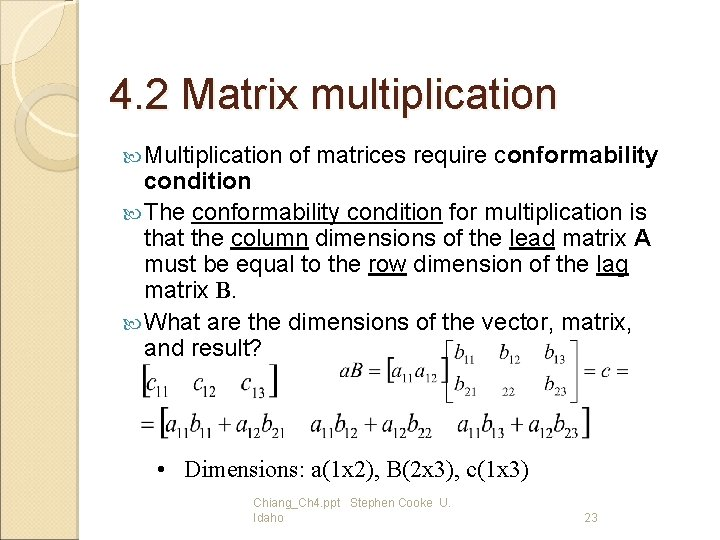4. 2 Matrix multiplication Multiplication of matrices require conformability condition The conformability condition for multiplication is that the column dimensions of the lead matrix A must be equal to the row dimension of the lag matrix B. What are the dimensions of the vector, matrix, and result? • Dimensions: a(1 x 2), B(2 x 3), c(1 x 3) Chiang_Ch 4. ppt Stephen Cooke U. Idaho 23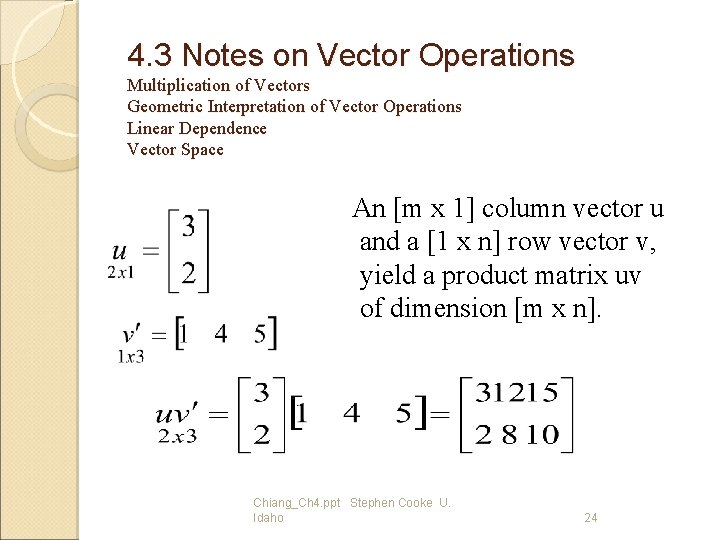4. 3 Notes on Vector Operations Multiplication of Vectors Geometric Interpretation of Vector Operations Linear Dependence Vector Space An [m x 1] column vector u and a [1 x n] row vector v, yield a product matrix uv of dimension [m x n]. Chiang_Ch 4. ppt Stephen Cooke U. Idaho 24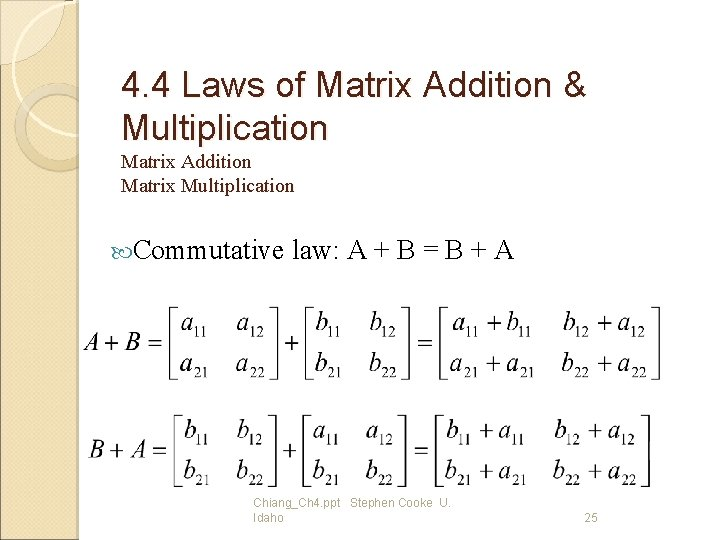4. 4 Laws of Matrix Addition & Multiplication Matrix Addition Matrix Multiplication Commutative law: A + B = B + A Chiang_Ch 4. ppt Stephen Cooke U. Idaho 254. 4 Matrix Multiplication Matrix multiplication is generally not commutative. That is, AB BA even if BA is conformable (because diff. dot product of rows or col. of A&B) Chiang_Ch 4. ppt Stephen Cooke U. Idaho 264. 7 Finite Markov Chains Markov processes are used to measure movements over time, e. g. , Example 1, p. 80 Chiang_Ch 4. ppt Stephen Cooke U. Idaho 274. 7 Finite Markov Chains associative law of multiplication Chiang_Ch 4. ppt Stephen Cooke U. Idaho 28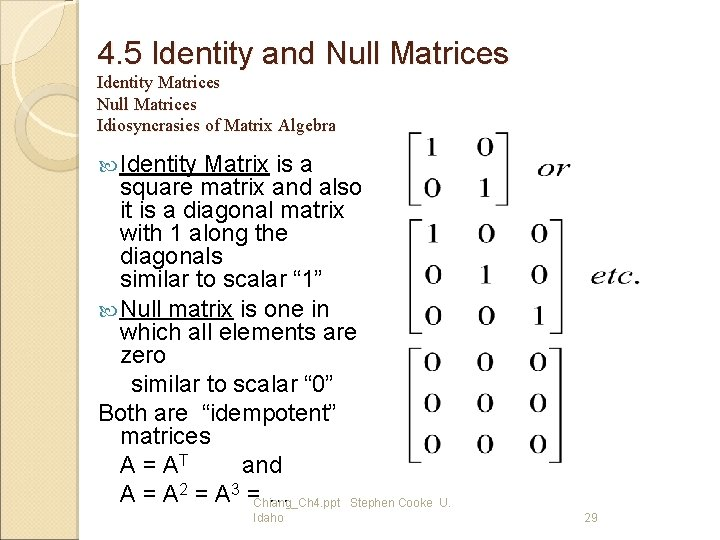4. 5 Identity and Null Matrices Identity Matrices Null Matrices Idiosyncrasies of Matrix Algebra Identity Matrix is a square matrix and also it is a diagonal matrix with 1 along the diagonals similar to scalar “ 1” Null matrix is one in which all elements are zero similar to scalar “ 0” Both are “idempotent” matrices A = AT and A = A 2 = A 3 = … Chiang_Ch 4. ppt Stephen Cooke U. Idaho 29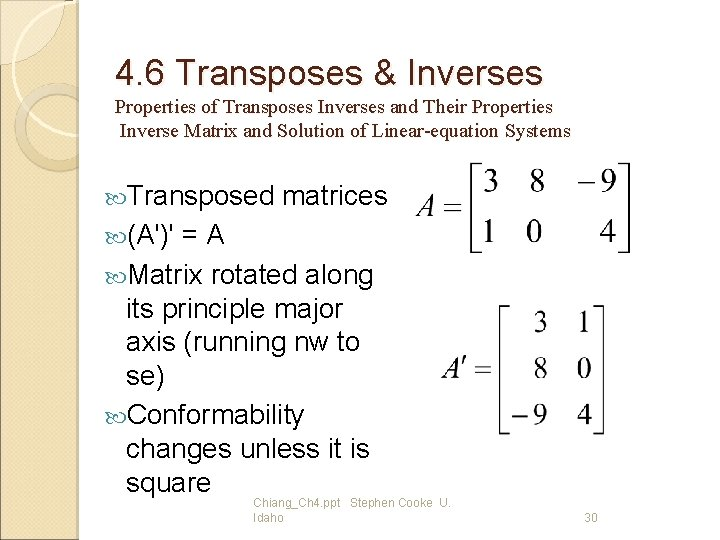4. 6 Transposes & Inverses Properties of Transposes Inverses and Their Properties Inverse Matrix and Solution of Linear-equation Systems Transposed matrices (A')' = A Matrix rotated along its principle major axis (running nw to se) Conformability changes unless it is square Chiang_Ch 4. ppt Stephen Cooke U. Idaho 304. 6 Inverse matrix • A-1 A=I • Necessary for • matrix to be square • to have inverse • If an inverse exists it is unique • (A')-1=(A-1)' • AA-1 = I Ax=d A-1 A x = A-1 d Ix = A-1 d Solution depends on A -1 Linear independence Determinant test! Chiang_Ch 4. ppt Stephen Cooke U. Idaho 314. 2 Matrix inversion It is not possible to divide one matrix by another. That is, we can not write A/B. This is because for two matrices A and B, the quotient can be written as AB-1 or B-1 A. • In matrix algebra AB-1 A. Thus writing does not clearly identify whether it represents AB-1 or B-1 A • Matrix division is matrix inversion • (topic of ch. 5) Chiang_Ch 4. ppt Stephen Cooke U. Idaho 32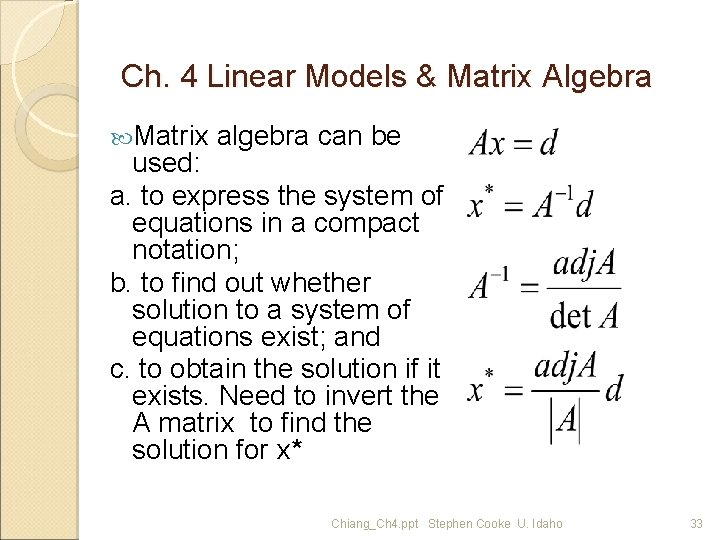Ch. 4 Linear Models & Matrix Algebra Matrix algebra can be used: a. to express the system of equations in a compact notation; b. to find out whether solution to a system of equations exist; and c. to obtain the solution if it exists. Need to invert the A matrix to find the solution for x* Chiang_Ch 4. ppt Stephen Cooke U. Idaho 33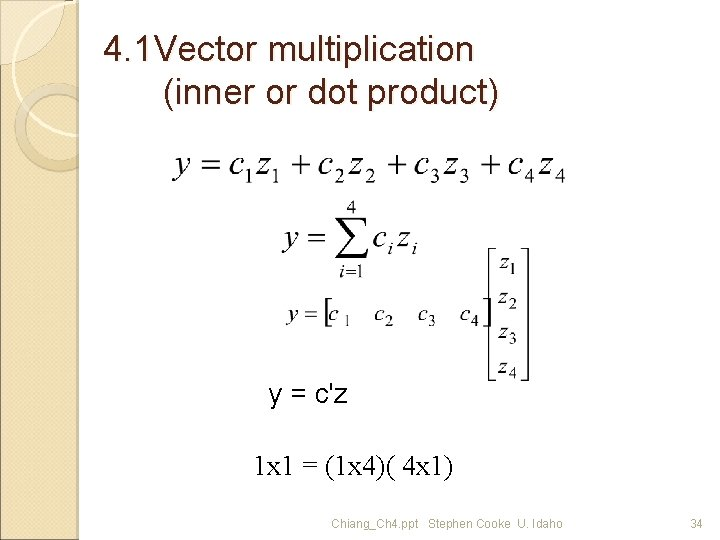4. 1 Vector multiplication (inner or dot product) y = c'z 1 x 1 = (1 x 4)( 4 x 1) Chiang_Ch 4. ppt Stephen Cooke U. Idaho 34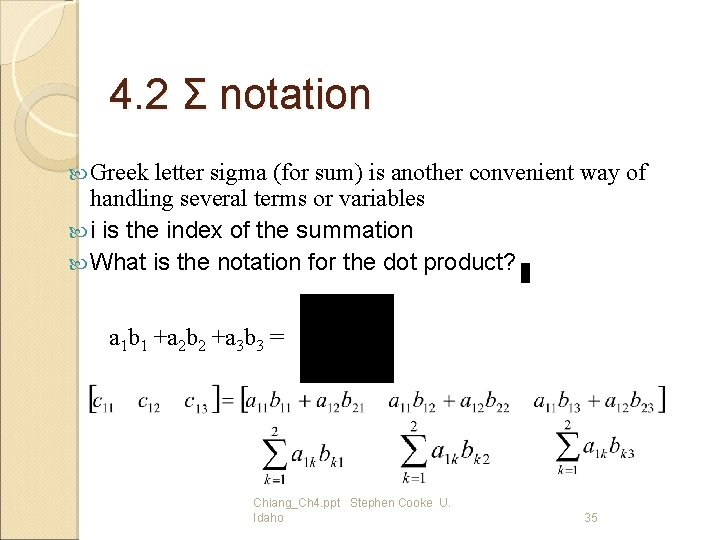4. 2 Σ notation Greek letter sigma (for sum) is another convenient way of handling several terms or variables i is the index of the summation What is the notation for the dot product? a 1 b 1 +a 2 b 2 +a 3 b 3 = Chiang_Ch 4. ppt Stephen Cooke U. Idaho 35